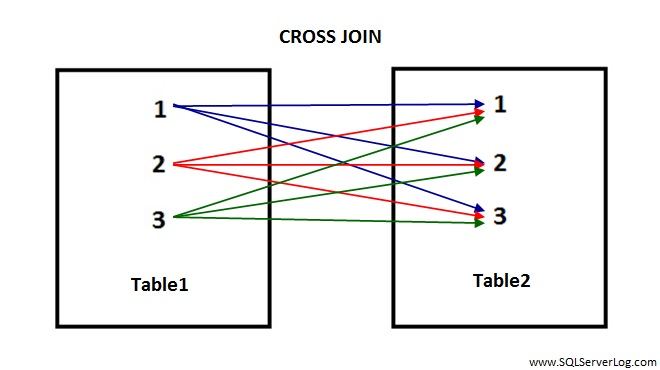# SQL CROSS JOIN

SQL CROSS JOIN returns all records where each row from the first table is combined with each row of the second table. CROSS JOIN returns the Cartesian product of the tables involved in join if WHERE clause is not used. The output of the Cartesian product is the number of rows from first table multiplied by the number of rows from second table.

## Syntax

SELECT * FROM

Table1 CROSS JOIN Table2

So, if there are 3 rows in table1 and 3 rows in table2 with CROSS JOIN then output without WHERE clause would be 3×3=9. So total 9 records will be returned by CROSS JOIN.You can refer above figure for CROSS JOIN and count color pointers from table1 to table2. As you can see with CROSS JOIN, each record from table1 is pointing to all records of table2.

## Also Refer:

Reference: Manzoor Siddiqui [www.SQLServerLog.com]